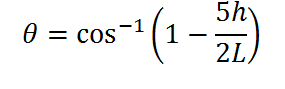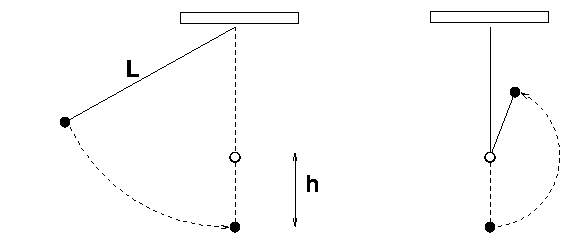# Height from which a pegged pendulum be dropped to complete the circle Calculator

This CalcTown calculator calculates the height from which the bob of a pendulum must be dropped so that after collision with a peg, it can complete the circle.

*If h>4L/5, then the bob will never complete the circle irrespective of the height it is dropped from.

m
m

#### Result

degreeClick here to view image

where

θ is the initial angle made by the pendulum with the vertical.

L is the length of the pendulum

h is the height of the peg from bottom